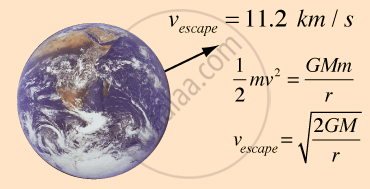# Escape Speed

#### notes

Escape Velocity

• Escape velocity is the minimum velocity that a body must attain to escape the gravitational field of the earth.

• Suppose if we throw a ball,it will fall back. This is happening due to the force of gravitation exerted on the ball by the surface of the earth due to which the ball is attracted towards the surface of the earth.

• If we increase the velocity to such an extent that the object which is thrown up will never fall back.This velocity is known as escape velocity.• Ball is thrown up but it falls down because of force of gravitation.
• The same ball is thrown with a velocity that it escapes the force of gravitation of earth and does not come back. This velocity is known as escape velocity.
• Mathematically:-

Suppose we throw a ball and the initial velocity of the ball is equal to the escape velocity such that ball never comes back.

Final Position will be infinity.

At Final Position: At Infinity

Total Energy (oo) = kinetic Energy (oo) + Potential Energy (oo)

Kinetic Energy (oo) = 1/2"mv"_f^2 where V_f=final velocity

Potential Energy (oo) = -"GMm"/"r" + V_o

Where M=mass of the earth, m= mass of the ball,

Vo=Potential energy at surface of earth, r=oo r= distance from the centre of the earth

therefore potential energy(oo)= 0

Total Energy(oo)=1/2"mv"_f^2        ...(1)

• At initial Position:

"E"=1/2"mv"_i^2

"E"=-("GMm")/(("R"_e + "h"))+"V"_o

Where h=height of the ball from the surface of the earth.

Total energy (initial) = 1/2mv_i^2 - "GMm"/(("R"_e+h))          ...(2)

accourding to the law of conservation of energy

Total energy(oo) = Toatal energy (initial)

1/2"m"v_f^2 = 1/2"m"v_i^2 - "GMm"/(("R"_e + "h"))

as LHS = Positive

1/2mv_i^2 - "GMm"/("R"_e + "h")>=0

By calculating

"v"_i^2 = "2GM"/(("R"_e + "h"))

Assume ball is thrown from the earths surface h << "R"_e

This implies "R"_e + "h" is same as Ras we can neglect h.

Therefore, "v"_i^2 = "2GM"/(("R"_e))

or "v"_i = sqrt("2GM"/"R"_e)

This is the initial velocity with which ifthe ball is thrown it will never fall back on the earths surface.

In terms of 'g'

"g" = "GM"/"R"_e^2

Escape velocity can be written as

"V"_e = sqrt"2gR"_e

Example of Escape velocity: No atmosphere on moon.

Earths escape velocity = "V"_e = sqrt"2gR"_e

Moons Escape velocity = "V"_e = sqrt(2"g"_m"R"_m) where "R"_m is the radius of the moon.

"g"_m = 1/6"g"_e; "R"_m = 1/4"R"_e

("V"_e)_"moon"=1/5("V"_e)_"earth"=2.3  "km"//s

As this velocity is very less, the molecules cannot accumulate on the moon so there is no atmosphere on the moon.

If you would like to contribute notes or other learning material, please submit them using the button below.

### Shaalaa.com

escape velocity [01:08:38]
S
0%